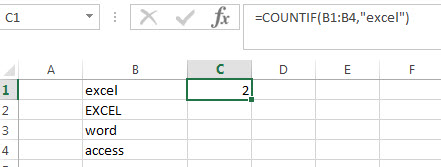# COUNTIF Function in Excel

This post will guide you how to use the COUNTIF function with syntax and examples in Microsoft excel.

## Description

The Excel COUNTIF function will count the number of cells in a range that meet a given criteria. This function can be used to count the different kinds of cells with number, date, text values, blank, non-blanks, or containing specific characters.etc.

we can also use the logical operators (>,>=,<,<=,<>,=) in Excel COUNTIF formula and also support wildcards characters (*,?) .

The COUNTIF function is a build-in function in Microsoft Excel and it is categorized as a Statistical Function.

The COUNTIF function is available in Excel 2016, Excel 2013, Excel 2010, Excel 2007, Excel 2003, Excel XP, Excel 2000, Excel 2011 for Mac.

## Syntax

The syntax of the COUNTIF function is as below:

`= COUNTIF (range, criteria)`

Where the COUNTIF function arguments are:
Range -This is a required argument.  The range of cells that you want to apply the criteria to count
Criteria – This is a required argument.  The criteria used to define which cells are counted

## COUNTIF Function Examples

The below examples will show you how to use Excel COUNTIF Function to return the number of cells in a range based on a given criteria.

Example1: Count the number of cells in range B1:B4 that contain “excel” text values`=COUNTIF(B1:B4,"excel")`

the above excel formula will return 2.

### Related Posts

• Count Cells That Contain Specific Text
This post will discuss that how to count the number of cells that contain specific text or certain text in a specified cells of range in Excel. How to get the total number of cells that contain certain text.……
• Count Cells That Contain  Text
If you want to count the number of cells that contain text, you can use the COUNTIF function and a wildcard to create a new Excel Formula. ……
• Highlight Cells That End with Specfic Characters
If you want to highlight cells that end with a specific text string, you can create a simple formula based on the COUNTIF function to apply conditional formatting. ……
• Finding the Max and Min value in an Alphanumeric Data
if you want to find the Maximal or minimal string value from an alphanumeric data list in the range B1:B5, you can create a formula based on the LOOKUP function and the COUNTIF function.….
• Count Cells between Two Dates with Multiple Criteria
Assuming that you have a list of data that contain two columns,  you want to count the number of product “excel” which is between two dates (from 2018/8/9-2018/10/19) in column B. You can create a new excel formula based on the SUMPRODUCT function to achieve the result.….
• COUNTIF with Multiple Criteria
To count the number based on multiple criteria in the same range or column in Excel, you can create a formula based on the SUMPRODUCT function and the COUNTIF function.….
• Count the Number of “Yes” / “No” in a Range
to count the number of “Yes” answer in the range of cells B2:B6 or count the number of “No” answer in the same range, and you need to create a formula based on the COUNTIF function.….
• Check If Multiple Cells are Equal In Excel
Assuming that you have a list of data in range A1:C1, and you want to compare if these cells are equal, if so, then return True, otherwise, return False. You need to create an Excel array formula based on the AND function and the EXACT function…
• Count Dates in Given Year/Month/Day
You can create a formula based on the SUMPRODUCT function and the YEAR function to count dates by a give year….
• Find Missing Numbers in a Sequence
You can use an excel array formula based on the SMALL function, the IF function, the ISNA function, the MATCH function, and the ROW function to find missing numbers in a sequence…
• Find Closest Value or Nearest Value in a Range
You need to use an excel array formula based on the INDEX function, the MATCH function, the MIN function and the ABS function to find Closest Value or Nearest Value in a Range in Excel…